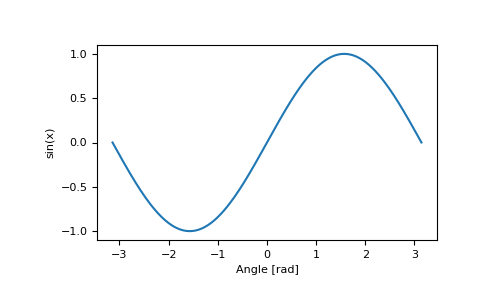#### Previous topic

Mathematical functions

numpy.cos

# numpy.sin¶

numpy.sin(x[, out]) = <ufunc 'sin'>

Trigonometric sine, element-wise.

Parameters: x : array_like Angle, in radians (2 \pi rad equals 360 degrees). y : array_like The sine of each element of x.

Notes

The sine is one of the fundamental functions of trigonometry (the mathematical study of triangles). Consider a circle of radius 1 centered on the origin. A ray comes in from the +x axis, makes an angle at the origin (measured counter-clockwise from that axis), and departs from the origin. The y coordinate of the outgoing ray’s intersection with the unit circle is the sine of that angle. It ranges from -1 for x=3\pi / 2 to +1 for \pi / 2. The function has zeroes where the angle is a multiple of \pi. Sines of angles between \pi and 2\pi are negative. The numerous properties of the sine and related functions are included in any standard trigonometry text.

Examples

Print sine of one angle:

>>> np.sin(np.pi/2.)
1.0


Print sines of an array of angles given in degrees:

>>> np.sin(np.array((0., 30., 45., 60., 90.)) * np.pi / 180. )
array([ 0.        ,  0.5       ,  0.70710678,  0.8660254 ,  1.        ])


Plot the sine function:

>>> import matplotlib.pylab as plt
>>> x = np.linspace(-np.pi, np.pi, 201)
>>> plt.plot(x, np.sin(x))